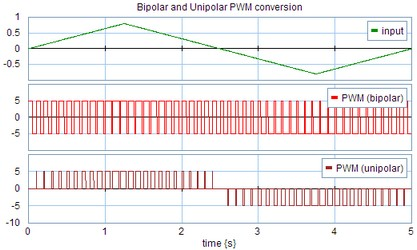﻿ 20-sim webhelp > Library > Signal > Block Diagram Non-Linear > signallimiter-pwm

# signallimiter-pwm

## Library

Signal\Block Diagram Non-Linear

## Use

Domains: Discrete, Continuous. Size: 1-D. Allowed in: Block Diagrams.

## Description

Pulse width modulation (PWM) is a powerful technique for controlling analog circuits with a processor's digital outputs. PWM is employed in a wide variety of applications, ranging from measurement and communications to power control and conversion. The output of a PWM block is a pulse train. The duty cycle, (the time that a pulse is on divided by the time the pulse is off) is proportional to the value of the input signal if the input is within the range < -input_amp , +input_amp >. For Bipolar PWM the output signal witches between a positive and a negative value. For Unipolar PWM the output signal witches between zero and positive or negative value.

 input bipolar PWM Unipolar PWM > input_amp 100% output_amp 0% -output_amp 100% output_amp 0%      0 input_amp 100% output_amp 0% -output_amp 100% output_amp 0%     0 0.5*input_amp 75% output_amp 25% -output_amp 50% output_amp 50% 0 0 50% output_amp 50% -output_amp 0 -0.5*input_amp 25% output_amp 75% -output_amp 50%  0 50% -output_amp -input_amp 0% output_amp 100% -output_amp 0%       0 100% -output_amp <-input_amp 0% output_amp 100% -output_amp 0%       0 100% -output_amp

An example is shown in the graph below. The parameter input_amp is equal to 1 and the parameter max output is equal to 5. The frequency of the PWM signal is 10 Hz.Bipolar and Unipolar PWM conversion.

## Interface

 Inputs Description input Outputs output Parameters f input_amp output_amp The modulating frequency [Hz]. Maximum value of the input signal. The output value will switch between output_amp, 0 and -output_amp.